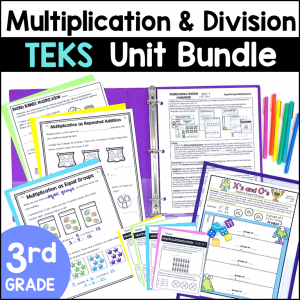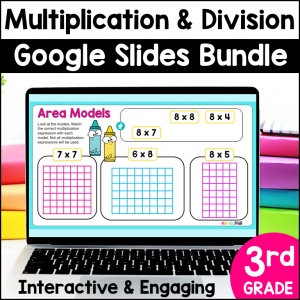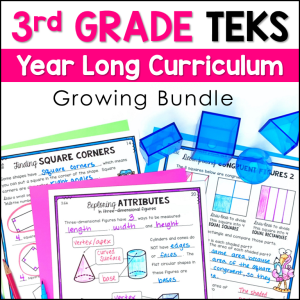Save 10% on your first order with code EXTRA10

# Arrays, Area Models and the Commutative Property of Multiplication

\$2.50\$54.25\$13.49\$296.75
SKU: 8541688 Category:

## Description

Your students will get fun & engaging practice with Arrays and Area Models while also learning about the Commutative Property of Multiplication. Students type multiplication equations for arrays and area models and then rotate the models by 90 degrees to see how this changes the equations. Students truly understand the commutative property with this interactive activity. This No Prep activity is perfect for 3rd grade students.

Video instructions for students are embedded in the activity, making it easy for you to assign as a no prep math station, for seatwork or homework.

This resource can be easily assigned in Google Classroom or any other platform compatible with Google Slides. Homeschool parents can use this resource by simply opening it in Google Slides. All you need to access Google Slides is a free Gmail account.

Save 35% by purchasing the Multiplication Foundations Google Slides Bundle!

What’s Included in this resource?

• PDF with a Link to make a copy of the Google Slides in your Google Drive
• Video Instructions (Student devices must be able to access You-Tube)
• 10 student practice slides
• Key

Topics Include:

• Arrays
• Area Models
• Multiplication Equations
• Commutative Property of Multiplication

Ideas on how to use this resource:

• Use it as a whole class teaching tool by projecting the slides and using the movable, interactive pieces to teach your lesson
• Use this resource as a no prep math station
• Use it as an early finisher activity
• Use this resource for small group reteach or tutoring
• Assign this resource as a lesson for student practice as independent work
• Share the digital key for parents and students to check work at home

Standards Alignment:

TEKS

3.4D Determine the total number of objects when equally sized groups of objects are combined or arranged in arrays up to 10 by 10

3.4K solve one‐step and two‐step problems involving multiplication and division within 100 using strategies based on objects; pictorial models, including arrays, area models, and equal groups; properties of operations; or recall of facts

Common Core

3.OA.A.3 Use multiplication and division within 100 to solve word problems in situations involving equal groups, arrays, and measurement quantities, e.g., by using drawings and equations with a symbol for the unknown number to represent the problem.

3.OA.B.5

Apply properties of operations as strategies to multiply and divide. Examples: If 6 × 4 = 24 is known, then 4 × 6 = 24 is also known. (Commutative property of multiplication.) 3 × 5 × 2 can be found by 3 × 5 = 15, then 15 × 2 = 30, or by 5 × 2 = 10, then 3 × 10 = 30. (Associative property of multiplication.) Knowing that 8 × 5 = 40 and 8 × 2 = 16, one can find 8 × 7 as 8 × (5 + 2) = (8 × 5) + (8 × 2) = 40 + 16 = 56. (Distributive property.)